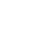## 背景

doT.js 6KB 4KB
mustache 18.9 KB 9.3 KB ×
Handlebars 512KB 62.3KB
artTemplate(腾讯) - 5.2KB
BaiduTemplate(百度) 9.45KB 6KB
jQuery-tmpl 18.6KB 5.98KB1. 小巧精简，源代码不超过两百行，6KB的大小，压缩版只有4KB；
2. 支持表达式丰富，涵盖几乎所有应用场景的表达式语句；
3. 性能优秀；
4. 不依赖第三方库。

## 如何使用

``````<script type="text/html" id="tpl">
<div>
<a>name:{{= it.name}}</a>
<p>age:{{= it.age}}</p>
<p>hello:{{= it.sayHello() }}</p>
<select>
{{~ it.arr:item}}
<option {{?item.id == it.stringParams2}}selected{{?}} value="{{=item.id}}">
{{=item.text}}
</option>
{{~}}
</select>
</div>
</script>
<script>
\$("#app").html(doT.template(\$("#tpl").html())({
name:'stringParams1',
stringParams1:'stringParams1_value',
stringParams2:1,
arr:[{id:0,text:'val1'},{id:1,text:'val2'}],
sayHello:function () {
return this[this.name]
}
}));
</script>
``````

## 源码分析及实现原理

1. 脱离后端渲染语言，不需要依赖后端项目的启动，从而降低了开发耦合度、提升开发效率；
2. View层渲染逻辑全在JavaScript层实现，容易维护和修改；
3. 数据通过接口得到，无需考虑后端数据模型变化，只需关心数据格式。

doT.js源码核心：

``````...
// 去掉所有制表符、空格、换行
str = ("var out='" + (c.strip ? str.replace(/(^|\r|\n)\t* +| +\t*(\r|\n|\$)/g," ")
.replace(/\r|\n|\t|\/\*[\s\S]*?\*\//g,""): str)
.replace(/'|\\/g, "\\\$&")
.replace(c.interpolate || skip, function(m, code) {
return cse.start + unescape(code,c.canReturnNull) + cse.end;
})
.replace(c.encode || skip, function(m, code) {
needhtmlencode = true;
return cse.startencode + unescape(code,c.canReturnNull) + cse.end;
})
// 条件判断正则匹配，包括if和else判断
.replace(c.conditional || skip, function(m, elsecase, code) {
return elsecase ?
(code ? "';}else if(" + unescape(code,c.canReturnNull) + "){out+='" : "';}else{out+='") :
(code ? "';if(" + unescape(code,c.canReturnNull) + "){out+='" : "';}out+='");
})
// 循环遍历正则匹配
.replace(c.iterate || skip, function(m, iterate, vname, iname) {
if (!iterate) return "';} } out+='";
sid+=1; indv=iname || "i"+sid; iterate=unescape(iterate);
return "';var arr"+sid+"="+iterate+";if(arr"+sid+"){var "+vname+","+indv+"=-1,l"+sid+"=arr"+sid+".length-1;while("+indv+"<l"+sid+"){"
+vname+"=arr"+sid+"["+indv+"+=1];out+='";
})
// 可执行代码匹配
.replace(c.evaluate || skip, function(m, code) {
return "';" + unescape(code,c.canReturnNull) + "out+='";
})
+ "';return out;")
...

try {
return new Function(c.varname, str);//c.varname 定义的是new Function（）返回的函数的参数名
} catch (e) {
/* istanbul ignore else */
if (typeof console !== "undefined") console.log("Could not create a template function: " + str);
throw e;
}
...
``````

### 代码解析重点1：正则替换

``````templateSettings: {
evaluate:    /\{\{([\s\S]+?(\}?)+)\}\}/g, //表达式
interpolate: /\{\{=([\s\S]+?)\}\}/g, // 插入的变量
encode:      /\{\{!([\s\S]+?)\}\}/g, // 在这里{{！不是用来做判断，而是对里面的代码做编码
use:         /\{\{#([\s\S]+?)\}\}/g,
useParams:   /(^|[^\w\$])def(?:\.|\[[\'\"])([\w\$\.]+)(?:[\'\"]\])?\s*\:\s*([\w\$\.]+|\"[^\"]+\"|\'[^\']+\'|\{[^\}]+\})/g,
define:      /\{\{##\s*([\w\.\$]+)\s*(\:|=)([\s\S]+?)#\}\}/g,// 自定义模式
defineParams:/^\s*([\w\$]+):([\s\S]+)/, // 自定义参数
conditional: /\{\{\?(\?)?\s*([\s\S]*?)\s*\}\}/g, // 条件判断
iterate:     /\{\{~\s*(?:\}\}|([\s\S]+?)\s*\:\s*([\w\$]+)\s*(?:\:\s*([\w\$]+))?\s*\}\})/g, // 遍历
varname:   "it", // 默认变量名
strip:    true,
append:       true,
selfcontained: false,
doNotSkipEncoded: false // 是否跳过一些特殊字符
}
``````

doT.js源码中对模板中语法正则替换的流程如下：### 代码解析重点2：new Function()运用

``````var funcName = new Function(p1,p2,...,pn,body);
``````

``````// 一般函数定义方式
function func1(a,b){
return a+b;
}
// 参数是一个字符串通过逗号分隔
var func2 = new Function('a,b','return a+b');
// 参数是多个字符串
var func3 = new Function('a','b','return a+b');
// 一样的调用方式
console.log(func1(1,2));
console.log(func2(2,3));
console.log(func3(1,3));
// 输出
3 // func1
5 // func2
4 // func3
``````

## 性能之因

#### jQuery-tmpl

``````function buildTmplFn( markup ) {
return new Function("jQuery","\$item",
// Use the variable __ to hold a string array while building the compiled template. (See https://github.com/jquery/jquery-tmpl/issues#issue/10).
"var \$=jQuery,call,__=[],\$data=\$item.data;" +

// Introduce the data as local variables using with(){}
"with(\$data){__.push('" +

// Convert the template into pure JavaScript
jQuery.trim(markup)
.replace( /([\\'])/g, "\\\$1" )
.replace( /[\r\t\n]/g, " " )
.replace( /\\$\{([^\}]*)\}/g, "{{= \$1}}" )
.replace( /\{\{(\/?)(\w+|.)(?:\(((?:[^\}]|\}(?!\}))*?)?\))?(?:\s+(.*?)?)?(\(((?:[^\}]|\}(?!\}))*?)\))?\s*\}\}/g,
function( all, slash, type, fnargs, target, parens, args ) {
//省略部分模板替换语句,若要阅读全部代码请访问:https://github.com/BorisMoore/jquery-tmpl
}) +
"');}return __;"
);
}
``````

with语句为什么对性能有这么大的影响？我们来看下面的代码：

``````var datas = {persons:['李明','小红','赵四','王五','张三','孙行者','马婆子'],gifts:['平民','巫师','狼','猎人','先知']};
function go(){
with(datas){
var personIndex = 0,giftIndex = 0,i=100000;
while(i){
personIndex = Math.floor(Math.random()*persons.length);
i--;
}
}
}
``````1. 模板缓存，在模板重复使用时，直接使用内存中缓存的模板。在本文作者看来，这是一个鸡肋的功能，在实际使用中，无论是直接写在String中的模板还是从Dom获取的模板都会以变量的形式存放在内存中，变量使用得当，在页面整个生命周期内都能取到这个模板。通过源码分析之后发现jQuery-tmpl的模板缓存并不是对模板编译结果进行缓存，并且会造成多次执行渲染时产生多次编译，再加上代码with性能消耗，严重拖慢整个渲染过程。
2. 模板标记，可以从缓存模板中取出对应子节点。这是一个不错的设计思路，可以实现数据改变只重新渲染局部界面的功能。但是我觉得：模板将渲染结果交给开发者，并渲染到界面指定位置之后，模板引擎的工作就应该结束了，剩下的对节点操作应该灵活的掌握在开发者手上。``````    function buildTmplFn( markup ) {

if(!compledStr){
// Convert the template into pure JavaScript
compledStr = jQuery.trim(markup)
.replace( /([\\'])/g, "\\\$1" )
.replace( /[\r\t\n]/g, " " )
.replace( /\\$\{([^\}]*)\}/g, "{{= \$1}}" )
.replace( /\{\{(\/?)(\w+|.)(?:\(((?:[^\}]|\}(?!\}))*?)?\))?(?:\s+(.*?)?)?(\(((?:[^\}]|\}(?!\}))*?)\))?\s*\}\}/g,
//省略部分模板替换语句
}

return new Function("jQuery","\$item",
// Use the variable __ to hold a string array while building the compiled template. (See https://github.com/jquery/jquery-tmpl/issues#issue/10).
"var \$=jQuery,call,__=[],\$data=\$item.data;" +

// Introduce the data as local variables using with(){}
"__.push('" + compledStr +
"');return __;"
)
}
``````

#### 仅25行的模板：tmpl

``````(function(){
var cache = {};

this.tmpl =  function (str, data){
var fn = !/\W/.test(str) ?
cache[str] = cache[str] ||
tmpl(document.getElementById(str).innerHTML) :

new Function("obj",
"var p=[],print=function(){p.push.apply(p,arguments);};" +
"with(obj){p.push('" +

str
.replace(/[\r\t\n]/g, " ")
.split("<%").join("\t")
.replace(/((^|%>)[^\t]*)'/g, "\$1\r")
.replace(/\t=(.*?)%>/g, "',\$1,'")
.split("\t").join("');")
.split("%>").join("p.push('")
.split("\r").join("\\'")
+ "');}return p.join('');");

return data ? fn( data ) : fn;
};
})();
``````## 总结

1. doT.js的条件判断语法标签不直观。当开发者在使用过程中条件判断嵌套过多时，很难找到对应的结束语法符号，开发者需要自己严格规范代码书写，否则会给开发和维护带来困难。
2. doT.js限制开发者自定义语法标签，相比较之下baiduTemplate提供可自定义标签的功能，而baiduTemplate的性能瓶颈恰好是提供自定义语法标签的功能。

## 作者简介